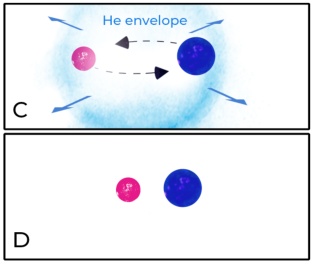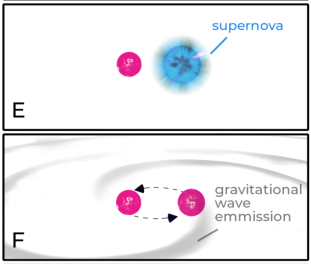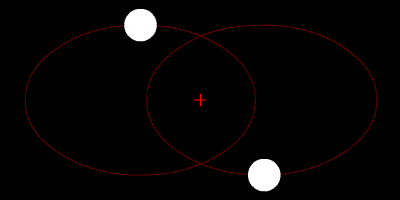GW190425 was the topic of my honours thesis and a subsequent paper by our team: Isobel Romero-Shaw, myself, Simon Stevenson, Eric Thrane, and Xing-Jiang Zhu.

Gravitational Waves from GW190425

Predicted in Albert Einstein’s general theory of relativity, gravitational waves are emitted by accelerating objects. Two stars orbiting one-another in a binary system will lose energy from the emission of gravitational waves, causing the separation between the two stars in the binary to decay over time. Additionally, if the binary is in a non-circular eliptical orbit, known as an eccentric orbit, the orbits will gradually become more circular over time. For two sufficiently close and dense compact objects, such as neutron stars or black holes, this leads to an inspiral where the stars become closer in an increasingly circular orbit.

On the 25th of April 2019, Advanced LIGO/Virgo made the second detection of gravitational waves (GW) originating from a double neutron star (DNS) inspiral. Advanced LIGO/Virgo observes gravitational waves by measuring the stretching and squeezing of space as the waves pass over two perpendicular 4km long tunnels. The gravitational wave, GW190425, is particularly interesting as it originated from an enormous double neutron star system.

The combined mass of GW190425 was inferred to be $$3.4^{+0.3}_{-0.1} M_{\odot}$$; that is 3.4 times the mass of our Sun whilst each star is roughly just ~$$10$$km across! The total mass of GW190425 is inconsistent with previously observed double neutron stars at a $$5\sigma$$ level, indicating that we may have just uncovered a new population.

Coalescing neutron stars in a simulation of GW190425, CoRe collaboration

The closer the stars get, the faster their orbital velocities become as they circle around each other. The orbital period (time taken for one revolution) decreases; reflected in the gravitational waves as an increasing frequency. The crescendo of the gravitational wave frequency is known as a chirp. The chirp ends with a merger between the two neutron stars, forming a supramassive neutron star or a black hole.

The sound of a chirp from two black holes colliding (GW150914 converted into sound waves, headphones recommended), LIGO

3.4 Times the Mass of our Sun?!

The observation of such a massive neutron star binary points us towards one of the most exciting questions in astrophysics: how do stellar binaries form and evolve? Currently, there are two predominant formation scenarios for compact binaries, which not only includes binary neutron star formation but also binary black holes and neutron star-black hole binaries.

In the dynamic formation scenario, two compact objects (neutron stars/black holes) form individually in a dense stellar environment such as a globular cluster, a collection of stars orbiting the core of a galaxy. These two compact objects are later brought together via dynamical interactions to form a binary.

In isolated binary evolution, two stars begin in an orbit where one of the stars begins to grow in size as it burns off the remainder of its hydrogen. The larger star may get too large and too close to the smaller star, causing its outer layer of hydrogen to be gravitationally pulled onto the smaller star, forming a common envelope of hydrogen that surrounds both stars. This mass transfer continues until the hydrogen layer of the larger star has been stripped off, leaving a helium star. Sometime later, this helium star will undergo a supernova explosion, collapsing into a neutron star. Later, the second star will also undergo a supernova and become a neutron star, leaving a binary neutron star.

The implied detection rate from GW190425 is higher than we expect from the dynamical channel, and the total mass is difficult to explain through standard isolated binary evolution. Therefore neither scenario fits well for explaining the origin of GW190425.

Possible Explanation: Unstable Case BB Mass TransferIllustration: Carl Knox

Unstable case BB mass transfer is a variation of isolated binary evolution. Following the standard scenario, a binary may consist of a primary neutron star with a high-mass Helium star companion (Panel A). Unstable case BB mass transfer occurs when Helium is pulled off the Heleium star onto the neutron star, forming a common envelope which now engulfs the neutron star and a remaining carbon-oxygen core (Panel C).Friction caused by the stars orbiting inside the common envelope transfers angular momentum to the surrounding helium, eventually ejecting the envelope leaving a tight binary orbit with an period of less than 1 hour (Panel D).

Supernova KicksSometime later the carbon oxygen core undergoes a supernova explosion (Panel E), collapsing into a second neutron star. During the supernova, the asymmetric distribution of mass in the collapse gives the neutron star a kick. This kick gives the star a boost in a random direction, leading to an elongated orbit with significant eccentricity.

Generally, supernova kicks are thought to disrupt many binaries, providing the star with an orbital velocity greater than its escape velocity. But this is not the case for unstable case BB binaries due to the helium envelope leaving a tightly bound orbit that is more likely to remain bound after a kick.

Explaining GW190425

Unstable case BB mass transfer is a great candidate for explaining the origin of GW190425 for several reasons:

1. Simulations from Ivanova et al. in 2003 showed that a heavy He star-NS binary can survive unstable case BB mass transfer, with one of their simulated binaries retaining a $$3.01 M_{\odot}$$ helium star after the common envelope.
2. For the more massive binaries, there is a higher chance for unstable case BB mass transfer to occur, which leads to a tightly bound orbit allowing these binaries to almost always survive the supernova kick due to their very tight orbit. Where high mass supernova in wider orbits are more likely to be disrupted.
3. The short orbital period of less than one hour makes these binaries unlikely to be detected in radio pulsar surveys, due to severe Doppler smearing. As well as a relatively short time to merger of less than 10 million years, which makes them unlikely to be detected before they merge when compared to other long-lived DNS. Perhaps explaining why we would not have observed DNS this heavy before.

Searching for Signs of Eccentricity

In our recent paper, we propose that GW190425 may have formed as an isolated binary via unstable case BB mass transfer. Where the first common envelope left a binary consisting of a $$\sim1.4M_{\odot}$$ neutron star and a $$\sim4-5M_{\odot}$$ helium star with an orbital period of $$0.1-2$$ days. The unstable case BB mass transfer may have accreted $$\sim0.05-0.1M_{\odot}$$ of mass onto the first born neutron star during the second common envelope. The second supernova ejected $$\sim1M_{\odot}$$ of mass leaving a $$\sim(1.4+2.0)M_{\odot}$$ DNS, likely with significant eccentricity as a result of the kick.

The eccentricity, $$e$$, describes the ellipticity of the orbit where $$e=0$$ is a circular orbit and the orbit becomes increasingly elongated as $$e\rightarrow1$$.Two equal masses orbiting a common barycenter with an eccentric orbit $$e>0$$.

To test our hypothesis, we measured the eccentricity of GW190425 using the LIGO/Virgo gravitational wave data so that we could compare it to expected eccentricities of simulated unstable case BB binaries.The main plot from our paper, showing probabilities of very small eccentricities ($$\log_{10}$$ scale) from the GW190425 signal and of simulated unstable case BB mass transfer binaries. Shown at a frequency of 10Hz.

It is possible that GW190425 formed through unstable case BB mass transfer. However we were not able to distinguish the small eccentricity of GW190425 as binaries that form through this channel have circularised to $$e<10^{-4}$$ by the time they’re detectable by Advanced LIGO/Virgo (a minimum frequency of 20Hz). Though we do find that future detectors which are able to detect gravitational waves at lower frequencies, such as the Cosmic Explorer and the space based LISA mission, will enable us to resolve these eccentricities and allow us to distinguish unstable case BB mass transfer binaries.

In a later post I hope to cover: some technical details of our method from Isobel Romero-Shaw, who led our team in measuring the eccentricity of GW190425; how we simulated eccentricities of unstable case BB mass transfer binaries; and some further prospects in measuring the eccentricity of merging neutron stars.

For more details and citations, see my honours thesis and the paper that our team (Isobel Romero-Shaw, myself, Simon Stevenson, Eric Thrane, Xing-Jiang Zhu) put together.

Updated: